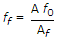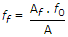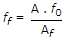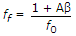# Electronics and Communication Engineering - Analog Electronics

21.

In a BJT amplifier circuit the size of coupling capacitor depends on

 A. lowest frequency B. highest frequency C. average of lowest and highest frequencies D. none of the above

Explanation:

No answer description available for this question. Let us discuss.

22.

In a closed loop non-inverting amplifier, constructed using single break-frequency op-amp, bandwidth with feedback can be written as. (f0 - break frequency, β - gain of the feedback circuit, A - open loop voltage gain, Af - closed loop voltage gain)

 A.B.C.D.Explanation:

No answer description available for this question. Let us discuss.

23.

A silicon sample A is doped with 1018 Atoms/cm3 of Boron. Another sample B of identical dimensions is doped with 1018 atoms/cm3 of phosphorus. The ratio of electron to hole mobility is 3. The ratio of conductivity of the sample A to B is

 A. 3 B. 1/3 C. 2/3 D. 3/2

Explanation:

No answer description available for this question. Let us discuss.

24.

Which of the following circuit has the greatest bandwidth?

 A. fr = 455 MHz; Q = 10 B. fr = 455 MHz; Q = 100 C. fr = 1 MHz; Q = 100 D. fr = 1 MHz; Q = 10

Explanation:

No answer description available for this question. Let us discuss.

25.

A FET is a __________ controlled device whereas a bipolar transistor is a __________ controlled device.

 A. gate, drain B. drain, gate C. current, voltage D. voltage, current